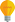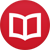# Pronunciation of the Greek alphabet in English

by Jakub MarianTip: See my list of theMost Common Mistakes in English. It will teach you how to avoid mis­takes with com­mas, pre­pos­i­tions, ir­reg­u­lar verbs, and much more.

Greek letters are widely used in mathematics and other fields of science. There are a couple of differences in pronunciation of the names of the letters between English and most other European languages, which is a common source of mistakes. That’s why in the following, I used a notation for pronunciation that should be easy to understand for non-native speakers, but native speakers should do just fine too.

Especially common mistakes are the names of $ι$, $μ$, $ν$ (which are not pronounced as yoh-tə, mee, and nee, respectively). Also, notice that $ξ$, $π$, $φ$, $χ$, and $ψ$ are pronounced with ‘eye’ at the end, not ‘ee’:

$α$ – alphaæl-fə
$β$ – betabee-tə (UK), bei-tə (US)
$γ$ – gamma-mə
$δ$ – deltadel-tə
$ε$ – epsiloneps-ill-ən or ep-sigh-lonn (UK), eps-ill-aan (US)
$ζ$ – zetazee-tə (UK), in the US more commonly zei-tə
$η$ – etaee-tə (UK), in the US more commonly ei-tə
$θ$ – thetathee-tə or thei-tə (in the US; both with ‘th’ as in think)
$ι$ – iota – I-oh-tə (‘I’ pronounced like ‘eye’)
$κ$ – kappa-pə
$λ$ – lambdalæm-də
$μ$ – mumyoo
$ν$ – nunyoo
$ξ$ – xiksaai (as in sick sigh) or zaai
$ο$ – omicron – oh-my-kronn (UK), aa-mə-kraan or oh-mə-kraan (US)
$π$ – pipaai (the same as pie)
$ρ$ – rhoroh (rhymes with go)
$σ$ – sigmasig-mə
$τ$ – tautaa’u (rhyming with cow) or taw (rhyming with saw)
$υ$ – upsilon‘ups’ as oops, ʌps or yoops, ‘ilon’ as ill-on or I’ll-ən
$φ$ – phifaai (as in identify)
$χ$ – chikaai (as in kite)
$ψ$ – psipsaai (as in top side) or saai (as in side)
$ω$ – omegaoh-meg-ə or oh-mɪg-ə (UK), oh-mey-gə or oh-meg(US)

By the way, if you haven’t read my guide on how to avoid the most common mistakes in English, make sure to check it out; it deals with similar topics.